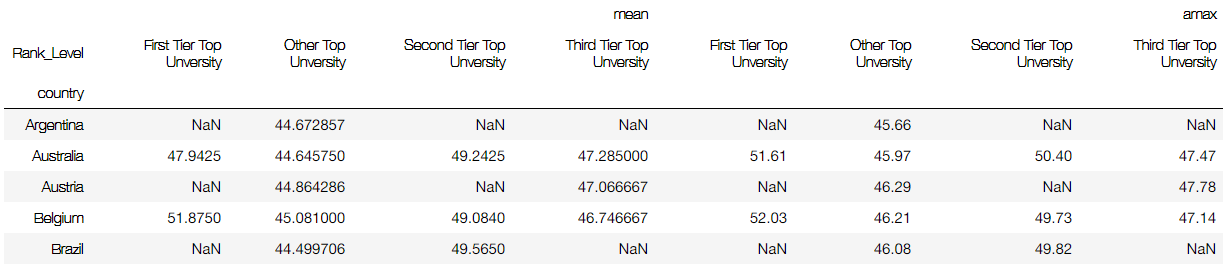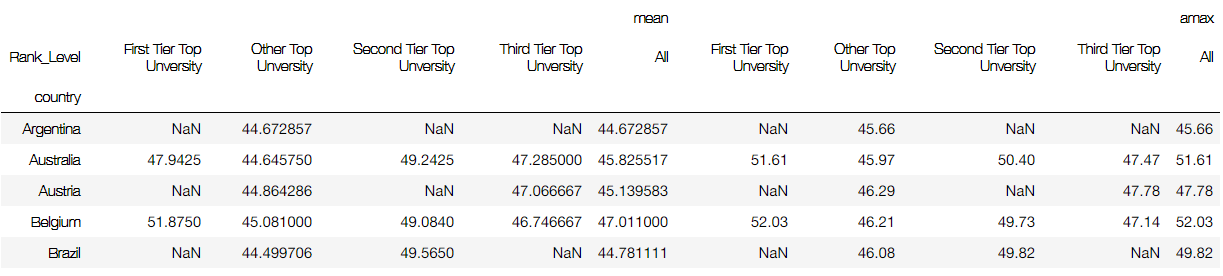" 有人相爱，有人夜里看海，有人巅峰赛九连败 "

# [Note3] IDSP-Pandas``````import pandas as pd
``````

# 1 Create

## 1.1 Structure

• Series
``````record1 = pd.Series({'Name': 'Alice',
'Class': 'Physics',
'Score': 85})
``````
``````s = pd.Series(['Physics', 'Chemistry', 'English'], index=['Alice', 'Jack', 'Molly'])
``````

• DataFrame
``````df = pd.DataFrame([record1, record2, record3],
index=['school1', 'school2', 'school1'])
``````
``````students = [{'Name': 'Alice',
'Class': 'Physics',
'Score': 85},
{'Name': 'Jack',
'Class': 'Chemistry',
'Score': 82},
{'Name': 'Helen',
'Class': 'Biology',
'Score': 90}]

# Then we pass this list of dictionaries into the DataFrame function
df = pd.DataFrame(students, index=['school1', 'school2', 'school1'])
``````

``````import pandas as pd

``````

Typically, opening excel files is much easier than csv due to different standard of .csv files. Some parameters can be useful when reading .csv

``````df = pd.read_csv('file.csv',sep=str, delimiter=str,header=int/list int,index_col=int, str,engine='C'/'python',)
``````

`sep/delimiter`
Delimiter to use. If `sep` is None, the C engine cannot automatically detect the separator, but the Python parsing engine can, meaning the latter will be used and automatically detect the separator by Python. In addition, separators longer than 1 character and different from '\s+' will be interpreted as regular expressions(re) and will also force the use of the Python parsing engine. Note that regex delimiters are prone to ignoring quoted data. Regex example: '\r\t'.

`header`

Row number(s) to use as the column names

`index_col`

`index_col=False` can be used to force pandas to not use the first column as the index, e.g. when you have a malformed file with delimiters at the end of each line.

## 1.3 Save

``````df.to_csv('file.csv')
df.to_excel('file.csv')
``````

# 2 Query

## 2.1 Basic

• Series
A pandas Series can be queried either by the index position or the index label. If you don't give an index to the series when querying, the position and the label are effectively the same values.
``````import pandas as pd
students_classes = {'Alice': 'Physics',
'Jack': 'Chemistry',
'Molly': 'English',
'Sam': 'History'}
s = pd.Series(students_classes)
``````
``````s.iloc
s.loc['Molly']
s
s['Molly']
``````

• DataFrame
``````df[df['chance of admit'] > 0.7]
df=df[df['SUMLEV'] == 50]

df[["gre score","toefl score"]]

columns_to_keep = ['STNAME','CTYNAME','BIRTHS2010','BIRTHS2011','BIRTHS2012','BIRTHS2013']
df = df[columns_to_keep]

df['SUMLEV'].unique()
``````

## 2.2 Boolean Musk

``````# Boolean masks are created by applying operators directly to the pandas Series or DataFrame objects.
# For instance, in our graduate admission dataset, we might be interested in seeing only those students
# that have a chance higher than 0.7

# To build a Boolean mask for this query, we want to project the chance of admit column using the
# indexing operator and apply the greater than operator with a comparison value of 0.7. This is
# essentially broadcasting a comparison operator, greater than, with the results being returned as
# a Boolean Series. The resultant Series is indexed where the value of each cell is either True or False
# depending on whether a student has a chance of admit higher than 0.7
``````

# 3 Indexing

## 3.1 reset and set index:

``````df = df.reset_index()
df = df.set_index(['STNAME', 'CTYNAME'])

#for query index and columns
df.index
df.columns
``````

## 3.2 rename columns

``````new_df=df.rename(columns={'GRE Score':'GRE Score', 'TOEFL Score':'TOEFL Score',
'University Rating':'University Rating',
'SOP': 'Statement of Purpose','LOR': 'Letter of Recommendation',
'CGPA':'CGPA', 'Research':'Research',
new_df.columns
new_df=new_df.rename(mapper=str.strip, axis='columns')
``````

## 3.3 overwrite columns name

``````cols = list(df.columns)
cols = [x.lower().strip() for x in cols]
# Then we just overwrite what is already in the .columns attribute
df.columns=cols
``````

# 4 Category

``````df["Grades"].astype("category").head()
my_categories=pd.CategoricalDtype(categories=['D', 'D+', 'C-', 'C', 'C+', 'B-', 'B', 'B+', 'A-', 'A', 'A+'],
ordered=True)
``````
``````df[df["Grades"]>"C"]
``````

Or you can create a method and apply it by lambda

``````def create_category(ranking):
# Since the rank is just an integer, I'll just do a bunch of if/elif statements
if (ranking >= 1) & (ranking <= 100):
return "First Tier Top Unversity"
elif (ranking >= 101) & (ranking <= 200):
return "Second Tier Top Unversity"
elif (ranking >= 201) & (ranking <= 300):
return "Third Tier Top Unversity"
return "Other Top Unversity"

# Now we can apply this to a single column of data to create a new series
df['Rank_Level'] = df['world_rank'].apply(lambda x: create_category(x))
# And lets look at the result
``````

# 4 Manipulation

## 4.1 Basic

``````(df.where(df['SUMLEV']==50)
.dropna()
.set_index(['STNAME','CTYNAME'])
.rename(columns={'ESTIMATESBASE2010': 'Estimates Base 2010'}))
``````

Second way is faster

``````df = df[df['SUMLEV']==50]
df.set_index(['STNAME','CTYNAME'], inplace=True)
df.rename(columns={'ESTIMATESBASE2010': 'Estimates Base 2010'})
``````

## 4.2 Apply function

``````def min_max(row):
data = row[['POPESTIMATE2010',
'POPESTIMATE2011',
'POPESTIMATE2012',
'POPESTIMATE2013',
'POPESTIMATE2014',
'POPESTIMATE2015']]
return pd.Series({'min': np.min(data), 'max': np.max(data)})

df.apply(min_max, axis=1)
df.apply(lambda x: np.max(x[rows]), axis=1)
``````

example:

``````def get_state_region(x):
northeast = ['Connecticut', 'Maine', 'Massachusetts', 'New Hampshire',
'Rhode Island','Vermont','New York','New Jersey','Pennsylvania']
midwest = ['Illinois','Indiana','Michigan','Ohio','Wisconsin','Iowa',
'South Dakota']
south = ['Delaware','Florida','Georgia','Maryland','North Carolina',
'South Carolina','Virginia','District of Columbia','West Virginia',
'Alabama','Kentucky','Mississippi','Tennessee','Arkansas',
'Louisiana','Oklahoma','Texas']

if x in northeast:
return "Northeast"
elif x in midwest:
return "Midwest"
elif x in south:
return "South"
else:
return "West"

df['state_region'] = df['STNAME'].apply(lambda x: get_state_region(x))
df[['STNAME','state_region']]
``````

# 5 Merge and Concatenate

## 5.1 Merge

``````pd.merge(staff_df, student_df, how='outer', left_index=True, right_index=True)
``````

`how=` 'inner'/'outer'/'left'/'right'

``````pd.merge(staff_df, student_df, how='right', on='Name')
pd.merge(staff_df, student_df, how='inner', on=['First Name','Last Name'])
``````

## 5.2 Concatenate

``````frames = [df_2011, df_2012, df_2013]
pd.concat(frames, keys=['2011','2012','2013'])
``````

# 6 Pivot table

A pivot table is a way of summarizing data in a DataFrame for a particular purpose. A pivot table also tends to includes marginal values as well, which are the sums for each column and row. This allows you to be able to see the relationship between two variables at just a glance.

``````df.pivot_table(values='score', index='country', columns='Rank_Level', aggfunc=[np.mean]).head()
````````````# So now we see we have both the mean and the max. As mentioned earlier, we can also summarize the values
# within a given top level column. For instance, if we want to see an overall average for the country for the
# mean and we want to see the max of the max, we can indicate that we want pandas to provide marginal values
df.pivot_table(values='score', index='country', columns='Rank_Level', aggfunc=[np.mean, np.max],
``````stack

``````df = new_df.stack()
df = df.unstack()
``````

• 267
• 0
0 0 vote
Article Rating
Subscribe0 评论
Inline Feedbacks